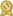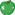Found 3778 calculus tutors – page 7 of 378.

Ahmedabad 320008 · \$20/hour · teaches Calculus, Geometry, GMAT, Math, Physics

Chemical Engineer M.B.M Engg College I have experience of more than 10 years in IIT JEE tutoring field. I am expert tutor for Test Prep like SAT, AP Calculus, AP Physics.Green Apple award, certified, member for 3 years and 9 months

Alexandria 22301 · \$80/hour · teaches Algebra 2 - Calculus - Geometry - Precalculus -

degree in applied mathematics in 2013 and have instructed on a wide range of mathematical courses in various branches of mathematics including Calculus I,II, Multivariable Calculus (Calculus 3), Advanced Calculus...certified

Plano 75024 · \$90/hour · teaches Algebra 2 - Calculus - Geometry - Physics -

University of Houston, Mathematics University of Houston, Masters Bejing University of Aeronautics and Astronautics, Masters I specialize in teaching calculus.certified

Fairport 14450 · \$65/hour · teaches Algebra 2 - Calculus - Geometry - Prealgebra -

At SUNY at Buffalo, I was an assistant for courses in calculus of a single variable, multivariable calculus, and linear algebra; at SUNY at Brockport, I was the instructor of a course in basic algebra.certified

Dayton 45458 · \$100/hour · teaches Algebra 2 - Calculus - Precalculus - Statistics

I am a patient tutor who enjoys breaking down concepts, solving business calculus problems, and helping students build confidence and enthusiasm for business calculus and mathematics in general.certified

Portland 97209 · \$50/hour · teaches Algebra 2 - Calculus - Geometry - Precalculus -

The majority of my three years as a math tutor was spent working with students taking calculus and helping them to make sense of their professor's and textbook's explanations that didn't make sense.certified

Bellevue 98008 · teaches Biology, Calculus, Chemistry, English, Grammar, Home Schooling

Club Z provides tutors with qualifications in the area of need. Our tutors are personable, well qualified and work with students one-on-one in homes.Green Apple award, member for 1 year and 8 months

Kennesaw 30160 · \$100/hour · teaches Algebra 2 - Calculus - Microsoft Excel - Geometry

I am a subject matter expert in the following: Algebra I, Algebra II, Geometry, Precalculus, Calculus I, Calculus II, Calculus III, AP Calculus AB, AP Calculus BC, ACT, SAT, GRE, Statistics, Data Analysis...certified

Gainesville 32607 · \$70/hour · teaches Algebra 2 - Calculus - Prealgebra - Precalculus

I specialize in Calculus and SAT Test Prep, although I am able to teach a wide array of subjects. In addition to my own teaching, I have worked alongside a test prep company to teach classes all over the world.certified

Granada Hills 91344 · \$75/hour · teaches Algebra 2 - Calculus - Geometry - Prealgebra -

I have taught and tutored many different mathematics courses including everything from Pre-Algebra to advanced Calculus. My favorite courses are Pre-Calculus, Algebra I, Geometry, Algebra II, Pre-Calculus...certified